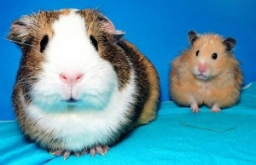# Pigs

A buyer said, "I want to buy 100 pigs for 100 denarii. An adult pig costs ten denarii, a sow five denarii, and two little piglets are worth one denarius. " How many pigs, cows, and piglets could he buy for exactly 100 denarii?

a =  1
b =  9
c =  90

### Step-by-step explanation:Did you find an error or inaccuracy? Feel free to write us. Thank you!

Tips for related online calculators
Do you have a linear equation or system of equations and looking for its solution? Or do you have a quadratic equation?
Do you solve Diofant problems and looking for a calculator of Diofant integer equations?